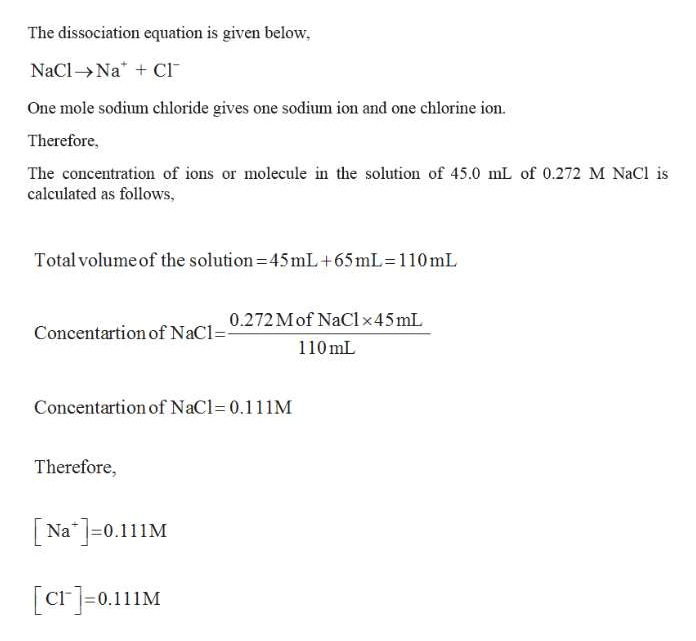# What is the concentration of ion or molecule in the solution of a mixture of 45.0 mL of 0.272 M NaCl and 65.0 mL of 0.0247 M (NH4)2CO3. Assume that the volume are additive.

Question
56 views
• What is the concentration of ion or molecule in the solution of a mixture of 45.0 mL of 0.272 M NaCl and 65.0 mL of 0.0247 M (NH4)2CO3. Assume that the volume are additive.

check_circle

Step 1

Given,

Solution of mixture of 45.0 mL of 0.272 M NaCl and 65.0 mL ...help_outlineImage TranscriptioncloseThe dissociation equation is given below, NaCl→Na* + CI One mole sodium chloride gives one sodium ion and one chlorine ion. Therefore, The concentration of ions or molecule in the solution of 45.0 mL of 0.272 M NaCl is calculated as follows, Total volume of the solution=45mL+65mL=110mL 0.272 Mof NaCl x45mL. 110 mL Concentartion of NaCl=- Concentartion of NaCl= 0.111M Therefore, [ Na"]=0.111M [cr]=0.11M fullscreen

### Want to see the full answer?

See Solution

#### Want to see this answer and more?

Solutions are written by subject experts who are available 24/7. Questions are typically answered within 1 hour.*

See Solution
*Response times may vary by subject and question.
Tagged in

### General Chemistry